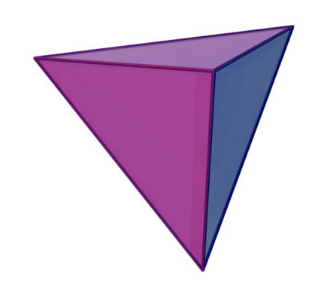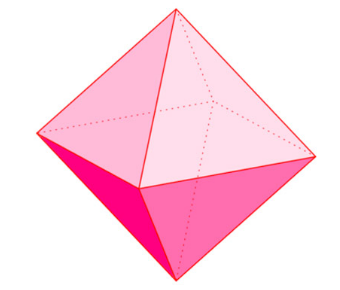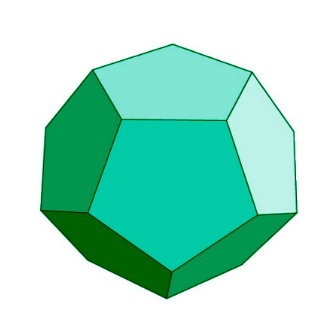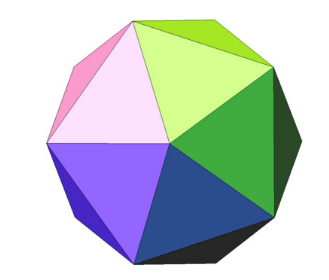# Platonic Solids Formula

Before getting to the formula, let us see the history of the name ”Platonic solids”. The ancient Greeks studied the Platonic solids pretty extensively. For the namesake, the platonic solids occur in the philosophy of Plato. Plato wrote about them in his book Timaeus c. 360 B.C. where he associated the four elements of Earth (earth, air, water and fire) with the regular solid. Cude was associated with The Earth, icosahedron was clubbed for water, and tetrahedron for fire.

The five platonic solids are:

• Tetrahedron
• Cube
• Octahedron
• Dodecahedron
• Icosahedron

They all are convex regular polyhedra. These five platonic solids have different formulas.

### FORMULA OF TETRAHEDRON

Tetrahedron: A tetrahedron has 4 faces, 4 vertices, 6 edges and 3 concurrent edges at a vertex:$\large Volume=\frac{\sqrt{2}}{12}\,a^{3}$

$\large Surface\;Area=\sqrt{3}\,a^{3}$

Cube: The cube is a solid which has has 6 faces, 8 vertices, 12 edges and 3 concurrent edges at a vertex:$\large Surface\;Area=4\,a^{2}$

$\large Volume=a^{3}$

$\large Diagonal=\sqrt{3}\:a$

Octahedron: A Solid which has 8 faces, 6 vertices, 12 edges and 4 concurrent edges at a vertex.$\large Surface\;Area=2\sqrt{3}\:a^{2}$

$\large Volume=\frac{\sqrt{2}}{3}\:a^{3}$

Dodecahedron: A solid which has 12 faces, 20 vertices, 30 edges and 3 concurrent edges at a vertex.$\large Surface\;Area=30\times a\times ap$

$\large Volume=\frac{1}{4}\left(15+7\sqrt{5}\right)a^{3}$

Icosahedron: A solid which has 20 faces, 12 vertices, 30 edges and 5 concurrent edges at a vertex.$\large Surface\;Area=5\sqrt{3}\:a^{2}$

$\large Volume=\frac{5}{12}\left(3+\sqrt{5}\:a^{3}\right)$